﻿ 基于改进乌鸦搜索算法的微电网多目标优化

# 基于改进乌鸦搜索算法的微电网多目标优化Multi-Objective Optimization of Microgrid Based on Improved Crow Search Algorithm

Abstract: In response to the national call for energy conservation and emission reduction, the distributed power output in the micro-grid is to be dispatched reasonably; while the lowest economic cost and the environmental cost are taken as the objective function, so as to establish mathematical models, as well as adopting the improved crow search algorithm (GCSA) to solve the model. Based on case simulation and compared with the traditional crow search algorithm (CSA), genetic algorithm (GA) and particle swarm optimization (PSO), it is verified that the proposed model and algorithm can ef-fectively reduce economic and environmental costs. In addition, the improved crow search algorithm (GCSA) has better optimization capabilities.

1. 引言

2. 微电网模型运行优化函数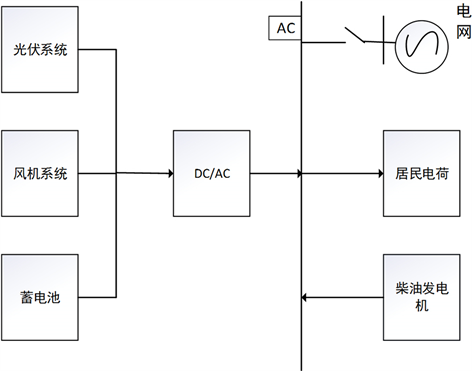Figure 1. Microgrid structure diagram

$\begin{array}{l}\mathrm{min}F\left(x\right)=\mathrm{min}\left({\left[{f}_{1}\left(x\right),{f}_{2}\left(x\right),\cdots ,{f}_{n}\left(x\right)\right]}^{\text{T}}\right)\\ \text{s}\text{.t}\text{.}\text{\hspace{0.17em}}\text{\hspace{0.17em}}\text{\hspace{0.17em}}x\in \text{Ω}\\ \text{\hspace{0.17em}}\text{\hspace{0.17em}}\text{\hspace{0.17em}}\text{\hspace{0.17em}}\text{\hspace{0.17em}}\text{\hspace{0.17em}}G\left(x\right)=0\\ \text{\hspace{0.17em}}\text{\hspace{0.17em}}\text{\hspace{0.17em}}\text{\hspace{0.17em}}\text{\hspace{0.17em}}\text{\hspace{0.17em}}H\left(x\right)\le 0\end{array}$ (1)

$F\left(x\right)=\mathrm{min}\left({\left[{f}_{c}\left(x\right),{f}_{e}\left(x\right)\right]}^{\text{T}}\right)$ (2)

${f}_{c}\left(x\right)={C}_{Ec}\left(x\right)+{C}_{Mn}\left(x\right)+{C}_{Fuel}\left(x\right)+{C}_{Bss}\left(x\right){\sum }_{i,pbss,i>0}^{H}{P}_{bss,i}$ (3)

${f}_{e}\left(x\right)={\sum }_{i=1}^{n}\left({V}_{e,i}{Q}_{i}\left(x\right)+{V}_{i}\right)$ (4)

3. 算法优化

3.1. 传统的乌鸦搜索算法

1) 乌鸦j不知道乌鸦i在后面跟随着，这是乌鸦 更新自己的位置为：

${x}^{i,iter+1}={x}^{i,iter}+{r}_{i}×f{l}^{i,iter}×\left({m}^{j,iter}-{x}^{i,iter}\right)\text{\hspace{0.17em}}\text{\hspace{0.17em}}\text{\hspace{0.17em}}{r}_{i}\gg AP$ (5)

2) 乌鸦j知道乌鸦i在后面跟随着，这时候乌鸦j会随机进入搜索空间的位置以此骗乌鸦i。

3.2. 改进的乌鸦搜索算法

$\left\{\begin{array}{l}{x}^{i,iter+1}={x}^{i,iter}+{r}_{i}×f{l}^{i,iter}×{\left({m}^{j,iter}-{x}^{i,iter}\right)}_{i}×f{l}^{i,iter}×\left({G}_{best}-{x}^{i,iter}\right)\text{\hspace{0.17em}}\text{\hspace{0.17em}}\text{\hspace{0.17em}}\text{\hspace{0.17em}}\text{\hspace{0.17em}}{r}_{i}\gg AP\\ \text{arandompositionotherwise}\end{array}$ (6)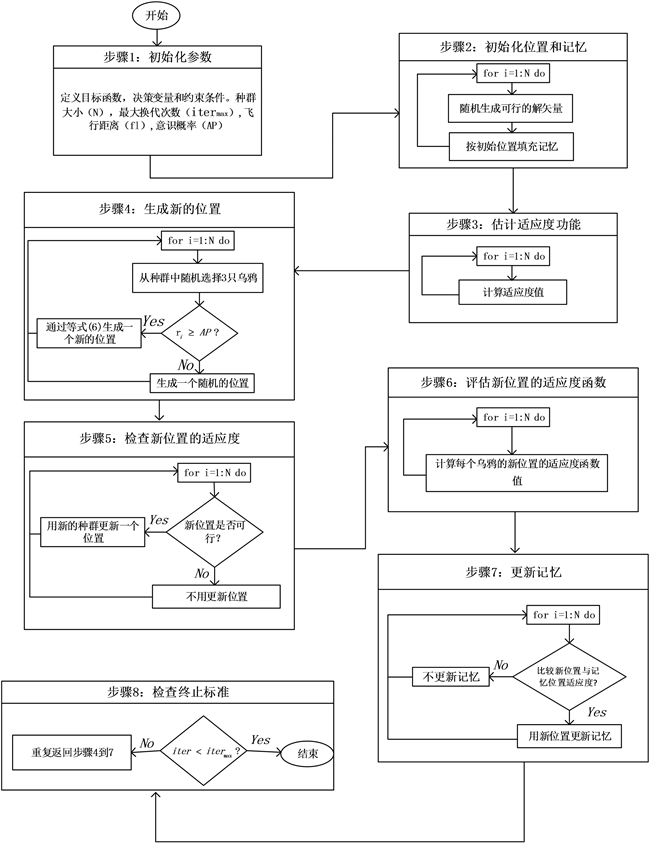Figure 2. GCSA algorithm flow chart

4. 算例分析

4.1. 算例基础数据Table 1. Parameters of distributed power sources in microgridTable 2. Pollutant treatment costs and emission coefficientsFigure 3. Residential load, PV, WT power change curveTable 3. Prices of electricity sales and purchases in each period

4.2. 孤岛算例分析

4.2.1. 风力(WT)涡轮机模型

${P}_{WT}=\left\{\begin{array}{l}0\text{\hspace{0.17em}}\text{\hspace{0.17em}}\text{\hspace{0.17em}}\text{\hspace{0.17em}}\text{\hspace{0.17em}}\text{\hspace{0.17em}}\text{\hspace{0.17em}}\text{\hspace{0.17em}}\text{\hspace{0.17em}}\text{\hspace{0.17em}}\text{\hspace{0.17em}}\text{\hspace{0.17em}}\text{\hspace{0.17em}}\text{\hspace{0.17em}}\text{\hspace{0.17em}}\text{\hspace{0.17em}}\text{\hspace{0.17em}}\text{\hspace{0.17em}}\text{\hspace{0.17em}}\text{\hspace{0.17em}}\text{\hspace{0.17em}}\text{\hspace{0.17em}}\text{\hspace{0.17em}}\text{\hspace{0.17em}}\text{\hspace{0.17em}}\text{\hspace{0.17em}}\text{\hspace{0.17em}}\text{\hspace{0.17em}}\text{\hspace{0.17em}}\text{\hspace{0.17em}}\text{\hspace{0.17em}}\text{\hspace{0.17em}}\text{\hspace{0.17em}}\text{\hspace{0.17em}}\text{\hspace{0.17em}}\text{\hspace{0.17em}}\text{\hspace{0.17em}}0\le u\le {u}_{i}\\ {P}_{WT,rate}\left(u-{u}_{i}\right)/\left({u}_{r}-{u}_{i}\right)\text{\hspace{0.17em}}\text{\hspace{0.17em}}\text{\hspace{0.17em}}\text{\hspace{0.17em}}\text{\hspace{0.17em}}\text{\hspace{0.17em}}\text{\hspace{0.17em}}{u}_{i}\le u\le {u}_{r}\\ {P}_{WT,rate}\text{\hspace{0.17em}}\text{\hspace{0.17em}}\text{\hspace{0.17em}}\text{\hspace{0.17em}}\text{\hspace{0.17em}}\text{\hspace{0.17em}}\text{\hspace{0.17em}}\text{\hspace{0.17em}}\text{\hspace{0.17em}}\text{\hspace{0.17em}}\text{\hspace{0.17em}}\text{\hspace{0.17em}}\text{\hspace{0.17em}}\text{\hspace{0.17em}}\text{\hspace{0.17em}}\text{\hspace{0.17em}}\text{\hspace{0.17em}}\text{\hspace{0.17em}}\text{\hspace{0.17em}}\text{\hspace{0.17em}}\text{\hspace{0.17em}}\text{\hspace{0.17em}}\text{\hspace{0.17em}}\text{\hspace{0.17em}}\text{\hspace{0.17em}}\text{\hspace{0.17em}}\text{\hspace{0.17em}}\text{\hspace{0.17em}}\text{\hspace{0.17em}}\text{\hspace{0.17em}}{u}_{r}\le u\le {u}_{0}\\ 0\text{\hspace{0.17em}}\text{\hspace{0.17em}}\text{\hspace{0.17em}}\text{\hspace{0.17em}}\text{\hspace{0.17em}}\text{\hspace{0.17em}}\text{\hspace{0.17em}}\text{\hspace{0.17em}}\text{\hspace{0.17em}}\text{\hspace{0.17em}}\text{\hspace{0.17em}}\text{\hspace{0.17em}}\text{\hspace{0.17em}}\text{\hspace{0.17em}}\text{\hspace{0.17em}}\text{\hspace{0.17em}}\text{\hspace{0.17em}}\text{\hspace{0.17em}}\text{\hspace{0.17em}}\text{\hspace{0.17em}}\text{\hspace{0.17em}}\text{\hspace{0.17em}}\text{\hspace{0.17em}}\text{\hspace{0.17em}}\text{\hspace{0.17em}}\text{\hspace{0.17em}}\text{\hspace{0.17em}}\text{\hspace{0.17em}}\text{\hspace{0.17em}}\text{\hspace{0.17em}}\text{\hspace{0.17em}}\text{\hspace{0.17em}}\text{\hspace{0.17em}}\text{\hspace{0.17em}}\text{\hspace{0.17em}}\text{\hspace{0.17em}}\text{\hspace{0.17em}}\text{\hspace{0.17em}}\text{\hspace{0.17em}}{u}_{0}\le u\end{array}$ (7)

${u}_{i}$ ——为切入风速；

${u}_{0}$ ——为切出风速；

${u}_{r}$ ——为额定风速。

${P}_{W{T}_{\mathrm{min}}}\ll {P}_{WT}\le {P}_{W{T}_{\mathrm{max}}}$ (8)

4.2.2. 光伏(PV)模型

${P}_{pv}={P}_{stc}\frac{{G}_{C}}{{G}_{STC}}\left[1+K\left({T}_{s}-{T}_{STC}\right)\right]$ (9)

${P}_{stc}$ ——标准条件下的最大输出功率；

${T}_{STC}$ ——参考温度；

${G}_{C}$ ——实际光照强度；

${T}_{S}$ ——工作温度；

K——功率温度系数。

${P}_{P{V}_{\mathrm{min}}}\ll {P}_{PV}\ll {P}_{P{V}_{\mathrm{max}}}$ (10)

4.2.3. 蓄电池(BSS)模型

$\left\{\begin{array}{l}SOC\left(t\right)=SOC\left(t-1\right)-{P}_{k}\left(t\right){\eta }_{c}{P}_{k}\left(t\right)\ge 0\\ SOC\left(t\right)=SOC\left(t-1\right)-{P}_{k}\left(t\right){\eta }_{d}{P}_{k}\left(t\right)\le 0\end{array}$ (11)

${p}_{k}\left(t\right)$ ——代表蓄电池的充放电功率( ${p}_{k}\left(t\right)\ge 0$ 代表充电状态、 ${p}_{k}\left(t\right)\le 0$ 代表放电状态)；

${\eta }_{c}$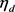——代表是充、放电效率。

${P}_{BS{S}_{\mathrm{min}}}\ll {P}_{BSS}\ll {P}_{BS{S}_{\mathrm{max}}}$ (12)

$SO{C}_{\mathrm{min}}\ll SOC\ll SO{C}_{\mathrm{max}}$ (13)

$SO{C}_{\mathrm{min}}$$SO{C}_{\mathrm{max}}$ 分别表示粗电池最小(取0.2)和最大(取0.8)剩余电量比例，SOC低于0.2不再放电、SOC高于0.8不再充电。

4.2.4. 柴油(DE)发电机模型

${C}_{DE}=\alpha +\beta {P}_{DE}+\gamma {P}_{DE}^{2}$ (14)

${P}_{DE}$ ——表示柴油发电机的发电功率；

$\alpha$$\beta$$\gamma$ ——表示燃料成本的系数。

${P}_{D{E}_{\mathrm{min}}}\ll {P}_{DE}\ll {P}_{D{E}_{\mathrm{max}}}$ (15)

${P}_{PV}+{P}_{WT}+{P}_{BSS}+{P}_{DE}={P}_{L}$ (16)

4.3. 结果分析

4.4. 算法比较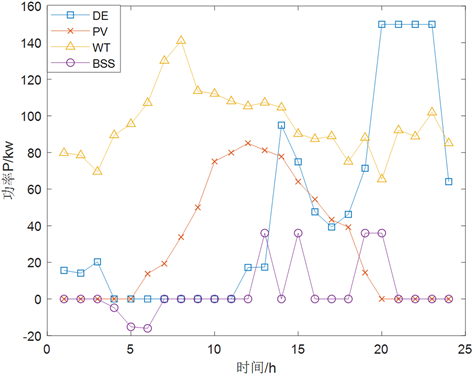Figure 4. The output power curve of each distributed power sourceFigure 5. Cost per hour

4.5. 并网算例分析

${P}_{PV}+{P}_{WT}+{P}_{BSS}+{P}_{DE}+{P}_{G}={P}_{L}$ (17)

${P}_{{G}_{\mathrm{min}}}\ll {P}_{G}\ll {P}_{{G}_{\mathrm{max}}}$ (18)

4.6. 结果分析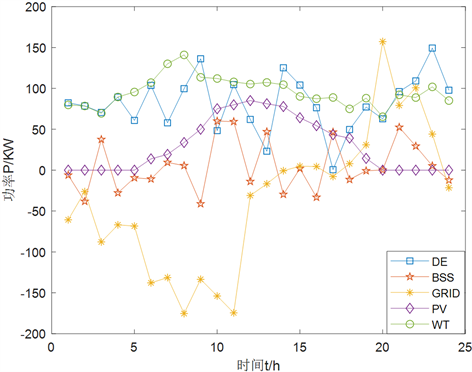Figure 6. The output power curve of each distributed power source

PV和WT为不可控力，出力应尽可能的多。从图6可以看出其中蓄电池在3:00、10:00、11:00、13:00、15:00、17:00以及20:00~23:00处于放电状态，缓解峰期的用电费用，在5:00和9:00处于既不放电也不充电的状态，其他时间段处于充电状态，为用电峰期做充足的准备。柴油发电机则弥补其余子系统与负载的差值部分。整个微电网运行期间，微电网在电价平期优先从电网购电，而其他系统的能量则储存在蓄电池中。

4.7. 算法比较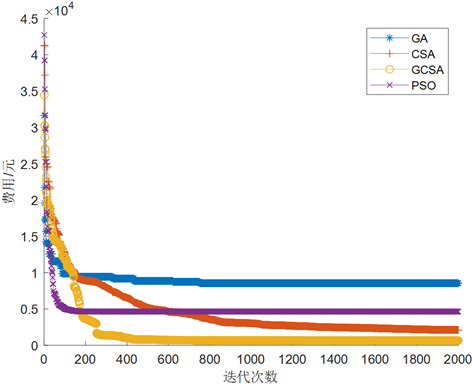Figure 7. Comparison of optimization capabilities of various algorithms

5. 结论

 Liu, M.X., et al. (2012) An Optimal Design Method of Muti-Objective Based Island Microgrid. Automation of Electric Power Systems, 17, 34-39.

 马溪原, 吴耀文, 方华亮, 等. 采用细菌觅食算法的风/光/储混合微电网电源优化配置[J]. 中国电机工程学报, 2011, 31(25): 17-25

 Sedighizadeh, M., Esmaili, M. and Eisapour-Moarref, A.J.A.S.C. (2017) Voltage and Frequency Regulation in Autonomous Microgrids Using Hybrid Big Bang-Big Crunch Algorithm. Applied Soft Computing, 52, 176-189.

 喻洁, 李扬, 夏安邦. 兼顾环境保护与经济效益的发电调度分布式优化策略[J]. 中国电机工程学报, 2009, 29(16): 63-8.

 刘盛松, 邰能灵, 侯志俭, 等. 基于最优潮流与模糊贴近度的电力系统环境保护研究[J]. 中国电机工程学报, 2003, 23(4): 21-26.

 赵磊, 曾芬钰. 孤岛运行下的微电网经济性优化研究[J]. 陕西电力, 2015, 43(7): 22-26.

 杨毅, 雷霞, 徐贵阳, 等. 采用PSO-BF算法的微电网多目标电能优化调度[J]. 电力系统保护与控制, 2014, 42(13): 49-55.

 张东, 陈景文, 张蓉, 等. 计及光伏预测的直流微电网能量调度研究[J]. 智慧电力, 2018, 46(2): 28-33.

 Morstyn, T., Hredzak, B. and Age-lidis, V. (2015) Distributed Cooperative Control of Microgrid Storage. IEEE Transactions on Power Systems, 30, 2780-2789.
https://doi.org/10.1109/TPWRS.2014.2363874

 Al-Qubaisi, A.M., Hssian, S. and Al-Alili, A. (2015) Optimization Based Fuzzy Resource Allocation Framework for Smart Grid. IEEE International Conference on Smart Energy Grid Engineering, Oshawa, ON, 1-6.

 卢锦玲, 苗雨阳, 张成相, 任惠. 基于改进多目标粒子群算法的含风电场电力系统优化调度[J]. 电力系统保护与控制, 2013(17): 25-31.

 Chaouachi, A., Kamelrm, R.M., Aadoulsi, R., et al. (2012) Multiobjective Intelligent Energy Management for a Microgrid. IEEE Transactions on Indus-trial Electronics, 60, 1688-1699.

 Rahbar, K., Xu, J. and Zhang, R.G. (2014) Real-Time Energy Storage Manage-ment for Renewable Integration in Microgrid: An Off-Line Optimization Approach. IEEE Transactions on Smart Grid, 6, 124-134.
https://doi.org/10.1109/TSG.2014.2359004

 Zhang, J., Wu, Y., Guo, Y., et al. (2016) A Hybrid Harmony Search Algorithm with Differential Evolution for Day-Ahead Scheduling Problem of a Microgrid with Consideration of Power Flow Constraints. Applied Energy, 183, 791-804.

Top